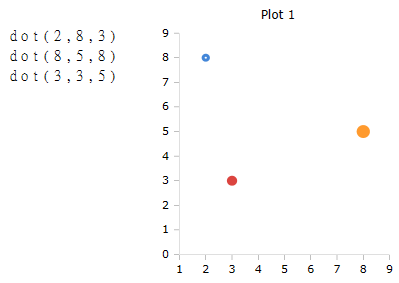# Dot Function

Draw a point in a plot box

### Description

The $$Dot$$ function draws a dot or ring in a plot box. Whether a filled dot or a ring is drawn depends on the specification of the parameter s and the size of the plot box.

### Syntax

Dot (x, y, s)

Dot (x, y, s, id)

### Parameters

x

The x position where the point is drawn.

y

The y position where the point is drawn.

s

The size of the point.

### Optional Parameters

id

Id number of the plot box, if more than one box is open on the worksheet.

### Example